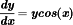### 1.5. Answers to Linear First Order Differential Equation Problems

Following are the answers to the practice questions presented throughout this chapter. Each one is worked out step by step so that if you messed one up along the way, you can more easily see where you took a wrong turn.

1 Is this equation a linear first order differential equation?Yes. This equation is a linear first order differential equation because it involves solely first order terms in y and y′.

2 Is the following a linear first order differential equation?No. This equation is not a linear first order differential equation because it doesn't involve solely first order terms in y and y′.

3 Is this equation a linear first order differential equation?No. This equation is not a linear first order differential equation because it doesn't involve solely first order terms in y and y′.

4 Is the following a linear first order differential equation?No. This equation is not a linear first order differential equation because it doesn't involve solely first order terms in y and y′.

5 Solve for y in this differential ...

Get Differential Equations Workbook For Dummies® now with the O’Reilly learning platform.

O’Reilly members experience live online training, plus books, videos, and digital content from nearly 200 publishers.